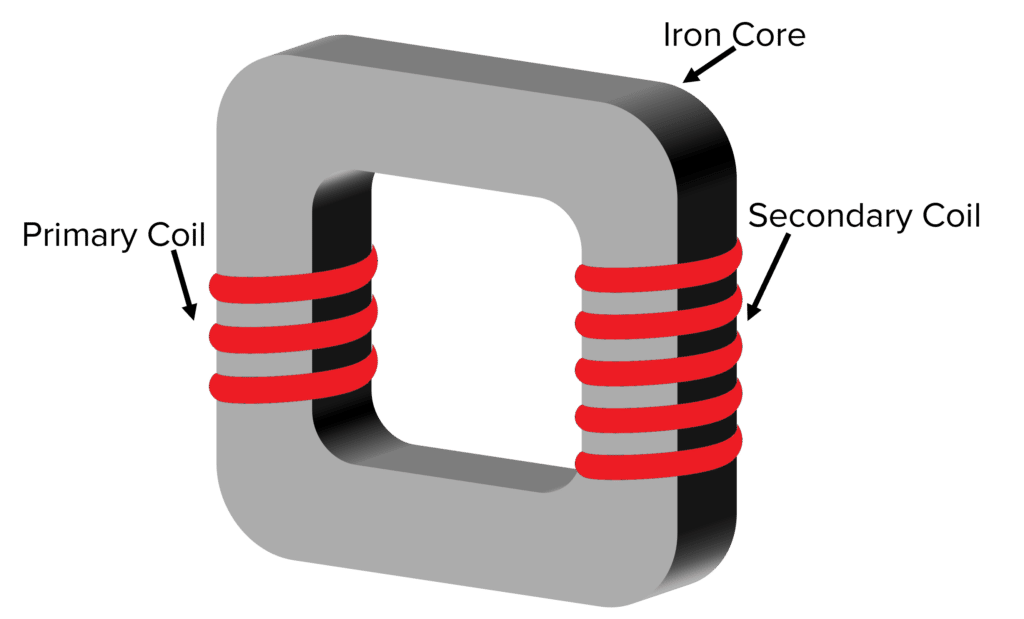# Transformers

GCSEAQACombined Science HigherPhysics Higher

## Transformers

Transformers change the voltage of alternating currents. They do this using electromagnetic induction, and are used in the national grid to change the voltage we use in our homes.

## Transformers

Transformers can increase or decrease the voltage of alternating currents. They do not work with direct currents.A transformer consists of two coils wound around an iron core. There is a primary coil and a secondary coil. The primary coil handles the input potential difference, and the secondary coil handles the output potential difference.

When an alternating potential difference is applied across the primary coil, the iron core will magnetise and then quickly demagnetise. An alternating potential difference is induced across the secondary coil due to this changing magnetic field. This is an example of electromagnetic induction.

• Step-up transformers have more turns on the secondary coil than the primary coil and therefore they increase the potential difference. A higher p.d means a lower current. This reduces energy loss via heat when the electricity is travelling through wires around the national grid.
• Step-down transformers have more turns on the primary coil than the secondary coil and they decrease the potential difference. They are used to reduce the voltage to a level that can be used in homes.
GCSECombined Science HigherPhysics HigherAQA

## The Transformer Equation

The ratio of the potential differences across the primary and secondary coils is dependent on the ratio of the number of turns on each coil. The transformer equation allows us to calculate the output potential difference of a transformer as long as we know the number of turns on each coil and the input potential difference:

$\textcolor{f21cc2}{\dfrac{V_p}{V_s} = \dfrac{n_p}{n_s}}$

• $\textcolor{f21cc2}{V_p}$ is the potential difference across the primary coil in volts $\left(V\right)$
• $\textcolor{f21cc2}{V_s}$ is the potential difference across the secondary coil in volts $\left(V\right)$
• $\textcolor{f21cc2}{n_p}$ is the number of turns on the primary coil
• $\textcolor{f21cc2}{n_s}$ is the number of turns on the secondary coil

For step-up transformers, $\textcolor{f21cc2}{V_s} > \textcolor{f21cc2}{V_p}$.

For step-down transformers, $\textcolor{f21cc2}{V_s} < \textcolor{f21cc2}{V_p}$.

GCSECombined Science HigherPhysics HigherAQA

## Transformers and Power

Transformers are not quite 100% efficient. However, they are very close. Therefore if we assume they are 100% efficient, then the electrical power output is equal to the electrical power input. This assumption allows us to use $P = VI$:

$\textcolor{00bfa8}{\text{electric power output} = \text{electric power input}}$

$\textcolor{00bfa8}{V_s I_s = V_p I_p}$

• $\textcolor{00bfa8}{V_p}$ is the input potential difference across the primary coil in volts $\left(V\right)$
• $\textcolor{00bfa8}{I_p}$ is the current through the primary coil in amps $\left(A\right)$
• $\textcolor{00bfa8}{V_s}$ is the output potential difference across the secondary coil in volts $\left(V\right)$
• $\textcolor{00bfa8}{I_s}$ is the current through the secondary coil in amps $\left(A\right)$
GCSECombined Science HigherPhysics HigherAQA

## Example: Transformer Calculations

A transformer has a primary coil with $\textcolor{bd0000}{50}$ turns and a secondary coil with $\textcolor{2730e9}{100}$ turns. The input potential difference is $\textcolor{00bfa8}{10 \: \text{V}}$.

Calculate the output potential difference in volts.

[3 marks]

We know the transformer equation is:

$\dfrac{V_p}{V_s} = \dfrac{n_p}{n_s}$

We want to find the output potential difference $V_s$. We can write the equation as:

$V_s = \dfrac{n_s \times V_p}{n_p}$

We know that $n_s = \textcolor{2730e9}{100}$, $n_p = \textcolor{bd0000}{50}$ and $V_p = \textcolor{00bfa8}{10 \: \text{V}}$, so we can substitute in the values:

$V_s = \dfrac{\textcolor{2730e9}{100} \times \textcolor{00bfa8}{10 \: \text{V}}}{\textcolor{bd0000}{50}}$

$V_s = 20 \: \text{V}$

GCSECombined Science HigherPhysics HigherAQA

## Transformers Example Questions

Step-up transformers are used to increase potential difference and step-down transformers are used to decrease the potential difference.

Step-up transformers have more turns on the secondary coil, whereas step-down transformers have more turns on the primary coil.Gold Standard Education

\begin{aligned} \boldsymbol{\dfrac{V_p}{V_s} }&\boldsymbol{= \dfrac{n_p}{n_s}} \\ \\ \boldsymbol{V_p} &\boldsymbol{= \dfrac{V_s \times n_p}{n_s}} \\ \\ V_p &= \dfrac{110 \times 6000}{2} \\ \\ \boldsymbol{V_s } &\boldsymbol{= 330000} \: \textbf{V} \end{aligned}Gold Standard Education

\begin{aligned} \boldsymbol{V_s I_s} &\boldsymbol{= V_p I_p} \\ \\ V_s &= \dfrac{V_p I_p}{I_s} \\ \\ \boldsymbol{V_s} &= \boldsymbol{\dfrac{10 \times 2}{4}} \\ \\ \boldsymbol{V_s} & \boldsymbol{= 5 \:} \textbf{V} \end{aligned}Gold Standard Education

## Transformers Worksheet and Example Questions

### Induced pd, Transformers and the National Grid Questions

GCSEOfficial MMEGold Standard Education

£19.99 /month. Cancel anytime